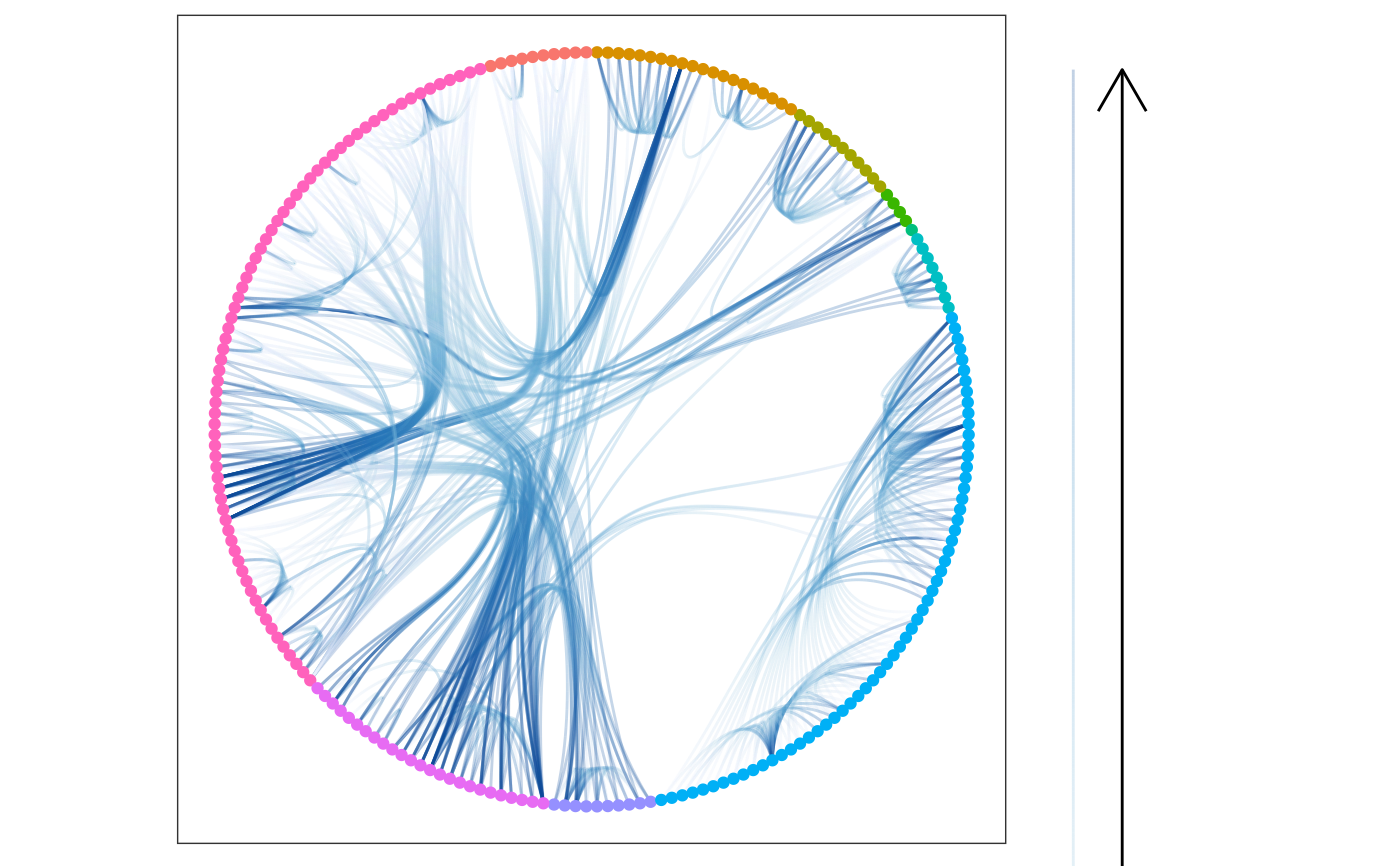Hierarchical edge bundling is a technique to introduce some order into the hairball structure that can appear when there's a lot of overplotting and edge crossing in a network plot. The concept requires that the network has an intrinsic hierarchical structure that defines the layout but is not shown. Connections between points (that is, not edges) are then drawn so that they loosely follows the underlying hierarchical structure. This results in a flow-like structure where lines that partly move in the same direction will be bundled together.

geom_conn_bundle(
mapping = NULL,
data = get_con(),
position = "identity",
arrow = NULL,
lineend = "butt",
show.legend = NA,
n = 100,
tension = 0.8,
...
)

geom_conn_bundle2(
mapping = NULL,
data = get_con(),
position = "identity",
arrow = NULL,
lineend = "butt",
show.legend = NA,
n = 100,
tension = 0.8,
...
)

geom_conn_bundle0(
mapping = NULL,
data = get_con(),
position = "identity",
arrow = NULL,
lineend = "butt",
show.legend = NA,
tension = 0.8,
...
)

## Arguments

mapping

Set of aesthetic mappings created by ggplot2::aes() or ggplot2::aes_(). By default x, y, xend, yend, group and circular are mapped to x, y, xend, yend, edge.id and circular in the edge data.

data

The result of a call to get_con()

position

Position adjustment, either as a string, or the result of a call to a position adjustment function.

arrow

Arrow specification, as created by grid::arrow().

lineend

Line end style (round, butt, square).

show.legend

logical. Should this layer be included in the legends? NA, the default, includes if any aesthetics are mapped. FALSE never includes, and TRUE always includes. It can also be a named logical vector to finely select the aesthetics to display.

n

The number of points to create along the path.

tension

How "loose" should the bundles be. 1 will give very tight bundles, while 0 will turn of bundling completely and give straight lines. Defaults to 0.8

...

Other arguments passed on to layer(). These are often aesthetics, used to set an aesthetic to a fixed value, like colour = "red" or size = 3. They may also be parameters to the paired geom/stat.

## Note

In order to avoid excessive typing edge aesthetic names are automatically expanded. Because of this it is not necessary to write edge_colour within the aes() call as colour will automatically be renamed appropriately.

## Aesthetics

geom_conn_bundle* understands the following aesthetics. Bold aesthetics are automatically set, but can be overridden.

• x

• y

• group

• circular

• edge_colour

• edge_width

• edge_linetype

• edge_alpha

• filter

## Computed variables

index

The position along the path (not computed for the *0 version)

## Author

Thomas Lin Pedersen

## Examples

# Create a graph of the flare class system
library(tidygraph)
flareGraph <- tbl_graph(flare$vertices, flare$edges) %>%
mutate(
class = map_bfs_chr(node_is_root(), .f = function(node, dist, path, ...) {
if (dist <= 1) {
return(shortName[node])
}
path$result[[nrow(path)]] }) ) #> Warning: Argument neimode' is deprecated; use mode' instead importFrom <- match(flare$imports$from, flare$vertices$name) importTo <- match(flare$imports$to, flare$vertices\$name)

# Use class inheritance for layout but plot class imports as bundles
ggraph(flareGraph, 'dendrogram', circular = TRUE) +
geom_conn_bundle(aes(colour = stat(index)),
data = get_con(importFrom, importTo),
edge_alpha = 0.25
) +
geom_node_point(aes(filter = leaf, colour = class)) +
scale_edge_colour_distiller('', direction = 1, guide = 'edge_direction') +
coord_fixed() +
ggforce::theme_no_axes()